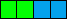# High-order word, low-order word

Sometimes some value can be stored in high-order word and low-order word of an integer value. I'll try to explain what it is.

At first you should know variable types int, word and byte.

int has 4 bytes. word has 2 bytes. Therefore int has 2 words.Green - low-order word.

Blue - high-order word.

The picture shows bytes in memory, in little-endian format, where the byte order is reverse than in hexadecimal representation (0xHHHHLLLL) and bit-shift operator names.

The low-order word adds its exact value to the total value of the int. The high-order word adds its value multiplied by 0x10000 (left-shifted by 16 bits).

To store two values L and H into low-order and high-order word of integer value R, use this:

```R = H<<16|L
or
R = MakeInt(L H)```

To get low-order word and high-order word:

```L = R&0xFFFF
H = R>>16```

### High-order byte, low-order byte

Sometimes some value can be stored in high-order byte and low-order byte of a word. Everything is similar as above.

To store two values L and H into low-order and high-order byte of word value R, use this:

`R = H<<8|L`

To get low-order byte and high-order byte:

```L = R&0xFF
H = R>>8&0xFF```